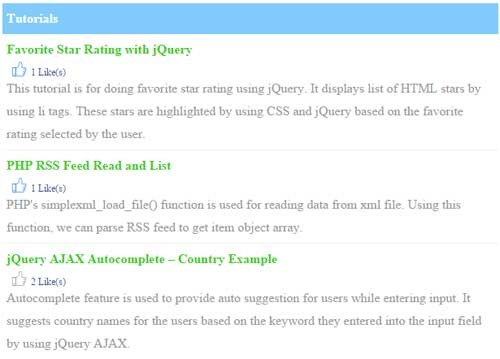# Facebook Style Like Unlike using PHP jQuery

This tutorial has an example code for doing like unlike which we have seen in many websites like Facebook. We are using jQuery AJAX to call PHP for updating likes count in the database.

We are using the IP address of the users to compute the like or unlike status.

## Database Results to Like Unlike

This code is for listing database results with like unlike icons based on the likes status.```<table class="demo-table">
<tbody>
<tr>
<th><strong>Tutorials</strong></th>
</tr>
<?php
if(!empty(\$result)) {
foreach (\$result as \$tutorial) {
?>
<tr>
<td valign="top">
<div class="feed_title"><?php echo \$tutorial["title"]; ?></div>
<div id="tutorial-<?php echo \$tutorial["id"]; ?>">
<input type="hidden" id="likes-<?php echo \$tutorial["id"]; ?>" value="<?php echo \$tutorial["likes"]; ?>">
<?php
\$query ="SELECT * FROM ipaddress_likes_map WHERE tutorial_id = '" . \$tutorial["id"] . "' and ip_address = '" . \$ip_address . "'";
\$count = \$db_handle->numRows(\$query);
\$str_like = "like";
if(!empty(\$count)) {
\$str_like = "unlike";
}
?>
<div class="btn-likes"><input type="button" title="<?php echo ucwords(\$str_like); ?>" class="<?php echo \$str_like; ?>" onClick="addLikes(<?php echo \$tutorial["id"]; ?>,'<?php echo \$str_like; ?>')" /></div>
<div class="label-likes"><?php if(!empty(\$tutorial["likes"])) { echo \$tutorial["likes"] . " Like(s)"; } ?></div>
</div>
<div class="desc"><?php echo \$tutorial["description"]; ?></div>
</td>
</tr>
<?php
}
}
?>
</tbody>
</table>
```

## jQuery Call for Like Unlike Update

This function will be called on the click event of like, unlike thumb icons. This will execute PHP code via AJAX and update database based on the requested action. On receiving successful AJAX response, this function will update the likes status and count. The script is,

```function addLikes(id,action) {
\$('.demo-table #tutorial-'+id+' li').each(function(index) {
\$('#tutorial-'+id+' #rating').val((index+1));
if(index == \$('.demo-table #tutorial-'+id+' li').index(obj)) {
return false;
}
});
\$.ajax({
data:'id='+id+'&action='+action,
type: "POST",
beforeSend: function(){
},
success: function(data){
var likes = parseInt(\$('#likes-'+id).val());
switch(action) {
case "like":
\$('#tutorial-'+id+' .btn-likes').html('<input type="button" title="Unlike" class="unlike" onClick="addLikes('+id+',\'unlike\')" />');
likes = likes+1;
break;
case "unlike":
\$('#tutorial-'+id+' .btn-likes').html('<input type="button" title="Like" class="like"  onClick="addLikes('+id+',\'like\')" />')
likes = likes-1;
break;
}
\$('#likes-'+id).val(likes);
if(likes>0) {
\$('#tutorial-'+id+' .label-likes').html(likes+" Like(s)");
} else {
\$('#tutorial-'+id+' .label-likes').html('');
}
}
});
}
```

## PHP MySQL Like Unlike Update Query Handling

This PHP code receives action from the AJAX call and performs database likes update based on this action. The code is,

```<?php
if(!empty(\$_POST["id"])) {
require_once("dbcontroller.php");
\$db_handle = new DBController();
switch(\$_POST["action"]){
case "like":
\$result = \$db_handle->insertQuery(\$query);
if(!empty(\$result)) {
\$query ="UPDATE tutorial SET likes = likes + 1 WHERE id='" . \$_POST["id"] . "'";
\$result = \$db_handle->updateQuery(\$query);
}
break;
case "unlike":
\$query = "DELETE FROM ipaddress_likes_map WHERE ip_address = '" . \$_SERVER['REMOTE_ADDR'] . "' and tutorial_id = '" . \$_POST["id"] . "'";
\$result = \$db_handle->deleteQuery(\$query);
if(!empty(\$result)) {
\$query ="UPDATE tutorial SET likes = likes - 1 WHERE id='" . \$_POST["id"] . "' and likes > 0";
\$result = \$db_handle->updateQuery(\$query);
}
break;
}
}
?>
```